# Lecture 9 Unconstrained Optimization Need to maximize a

• Slides: 31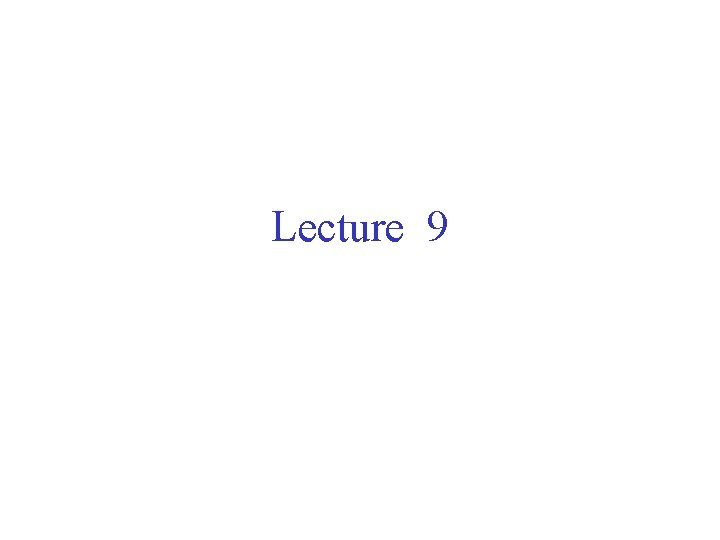Lecture 9Unconstrained Optimization Need to maximize a function f(x), where x is a scalar or a vector x = (x 1, x 2) f(x) = -(x-a)2 f(x) = x f(x) = -x 12 - x 22Gradient Search Techniques Suppose f(x) is differentiable, i. e. , f(x) exists. Then the series of updates xk+1 = xk + f(xk) reaches a local maxima starting from any initial value x 0, for all sufficiently small values of . A function f(x) has a local maxima at xk if f(xk) is less than or equal to f(y) for all y in some small neighborhood of xkConcave Function Let C be a convex subset of Rn A function f: C R is called concave if f( x + (1 - )y) f(x) + (1 - )f(y) for all [0, 1] and x , y C f(x) f( x + (1 - )y) f(x) + (1 - )f(y) x y x + (1 - )y xStrict Concave Function Let C be a convex subset of Rn A function f: C R is called strictly concave if f( x + (1 - )y) f(x) + (1 - )f(y) for all [0, 1] and x , y C A straight line is concave, but not strictly concave. Any local maxima is a global maxima for a concave function. A strictly concave function has a unique global maxima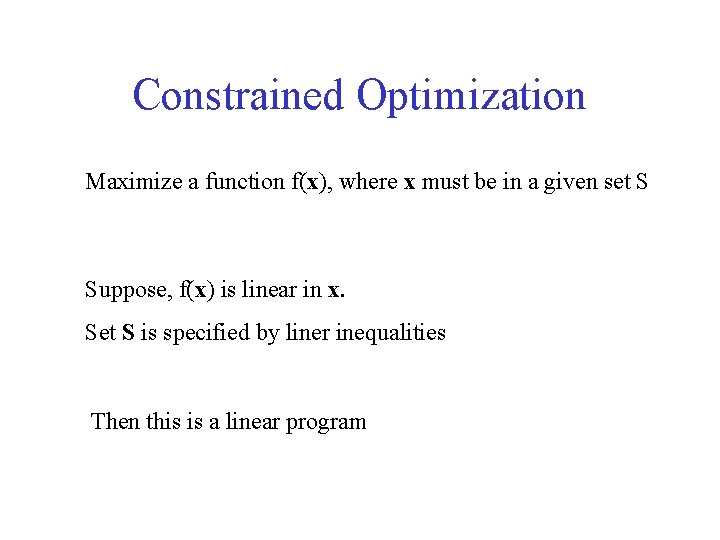Constrained Optimization Maximize a function f(x), where x must be in a given set S Suppose, f(x) is linear in x. Set S is specified by liner inequalities Then this is a linear program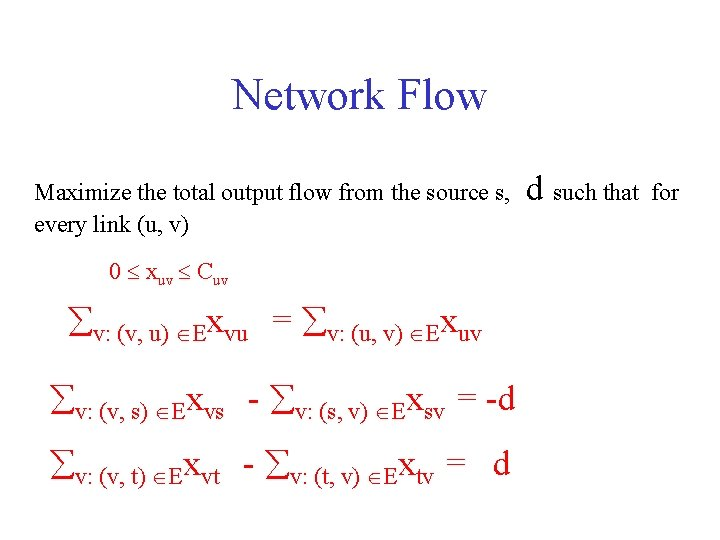Network Flow Maximize the total output flow from the source s, every link (u, v) 0 xuv Cuv v: (v, u) Exvu = v: (u, v) Exuv v: (v, s) Exvs - v: (s, v) Exsv = -d v: (v, t) Exvt - v: (t, v) Extv = d d such that for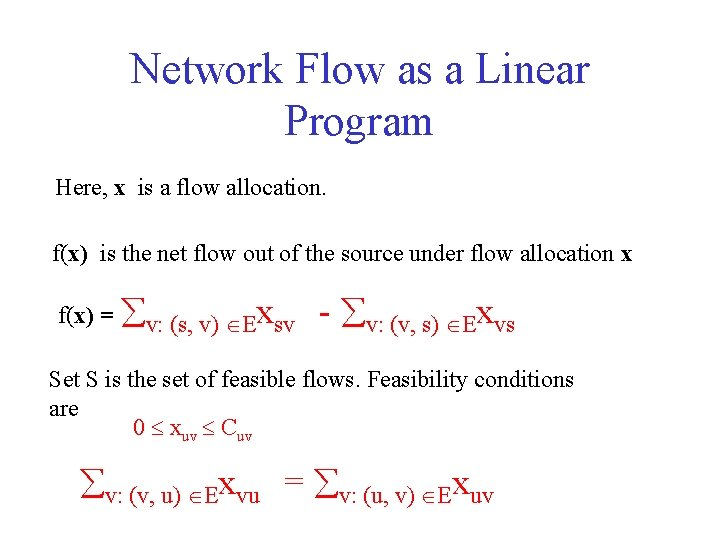Network Flow as a Linear Program Here, x is a flow allocation. f(x) is the net flow out of the source under flow allocation x f(x) = v: (s, v) Exsv - v: (v, s) Exvs Set S is the set of feasible flows. Feasibility conditions are 0 xuv Cuv v: (v, u) Exvu = v: (u, v) ExuvLinear Program. Techniques Simplex Exponential Complexity in the worst case However, LP is polynomial complexity computable. If a problem can be modelled as LP, then this shows that the problem is polynomial complexity and not NP-hard.Convex Optimization Maximize a function f(x), where x must be in a given set S Function f(x) is concave Set S is convex Get Rid of the constraints! Consider a function g(x) such that g(x) is small if x is not in S and large otherwise. Now try to maximize f(x) + g(x) without any constraints Now f(x) + g(x) can be maximized by gradient search techniques if f(x) + g(x) is differentiable.Let us be more specific about set S. A vector x belongs to set S if gi(x) 0, i=1, …M We would like to maximize f(x) - i gi(x) What should be the value of ? q( ) = maxx (f(x) - i gi(x)) min 0 q( ) The output of the above minimization is the maximum value of f(x) subject to x S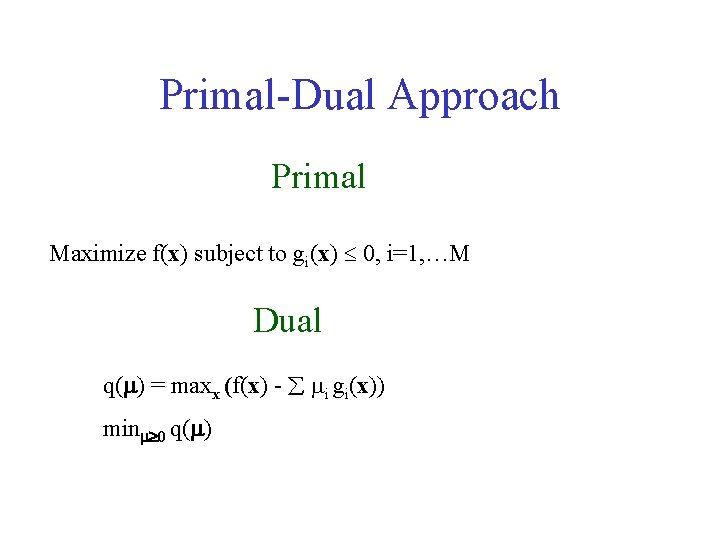Primal-Dual Approach Primal Maximize f(x) subject to gi(x) 0, i=1, …M Dual q( ) = maxx (f(x) - i gi(x)) min 0 q( )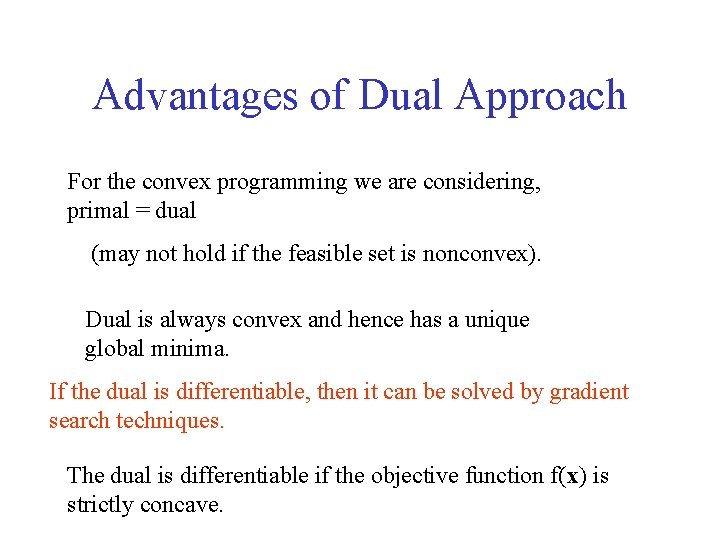Advantages of Dual Approach For the convex programming we are considering, primal = dual (may not hold if the feasible set is nonconvex). Dual is always convex and hence has a unique global minima. If the dual is differentiable, then it can be solved by gradient search techniques. The dual is differentiable if the objective function f(x) is strictly concave.Flow Control Sessions are normally greedy. Would like to send as much traffic as possible However, this would congest the network. Need to regulate the flow of the sessions. Flow of a session must depend on the bandwidth requirements and the revenues paid by the sessions.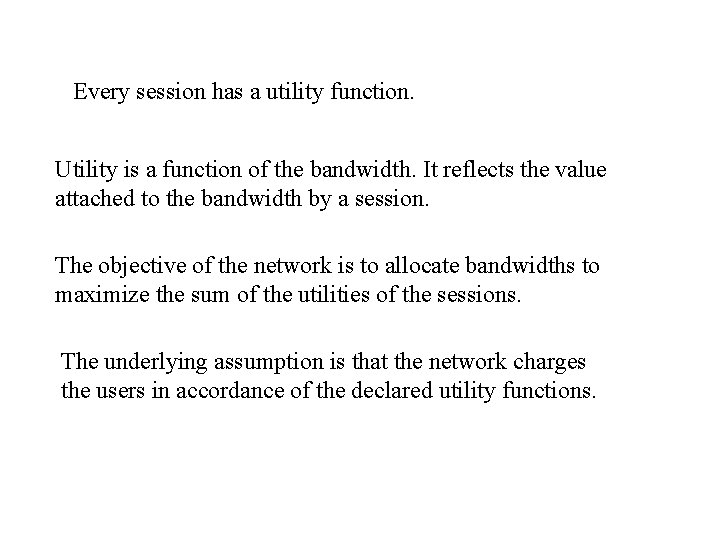Every session has a utility function. Utility is a function of the bandwidth. It reflects the value attached to the bandwidth by a session. The objective of the network is to allocate bandwidths to maximize the sum of the utilities of the sessions. The underlying assumption is that the network charges the users in accordance of the declared utility functions.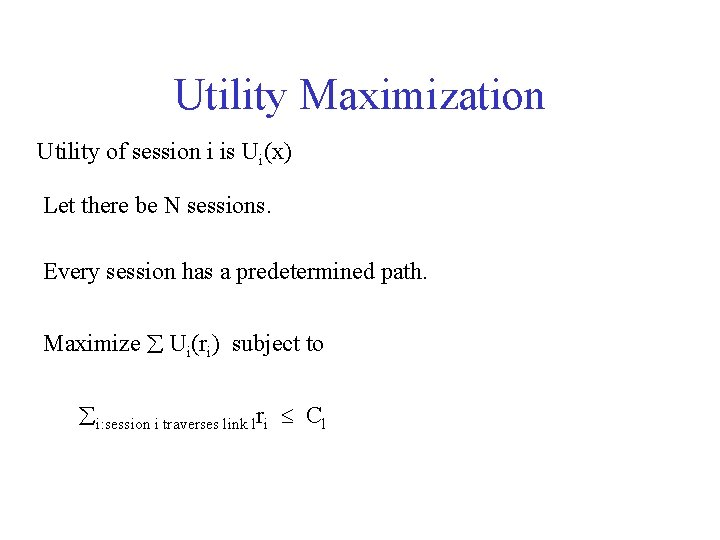Utility Maximization Utility of session i is Ui(x) Let there be N sessions. Every session has a predetermined path. Maximize Ui(ri) subject to i: session i traverses link lri Cl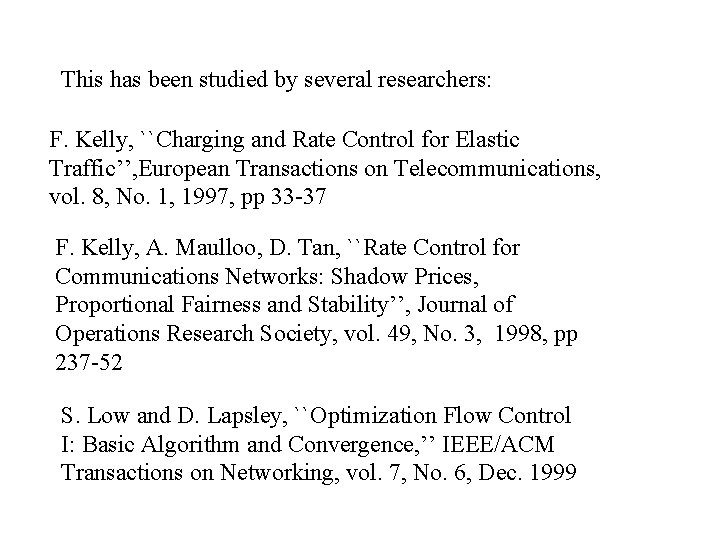This has been studied by several researchers: F. Kelly, ``Charging and Rate Control for Elastic Traffic’’, European Transactions on Telecommunications, vol. 8, No. 1, 1997, pp 33 -37 F. Kelly, A. Maulloo, D. Tan, ``Rate Control for Communications Networks: Shadow Prices, Proportional Fairness and Stability’’, Journal of Operations Research Society, vol. 49, No. 3, 1998, pp 237 -52 S. Low and D. Lapsley, ``Optimization Flow Control I: Basic Algorithm and Convergence, ’’ IEEE/ACM Transactions on Networking, vol. 7, No. 6, Dec. 1999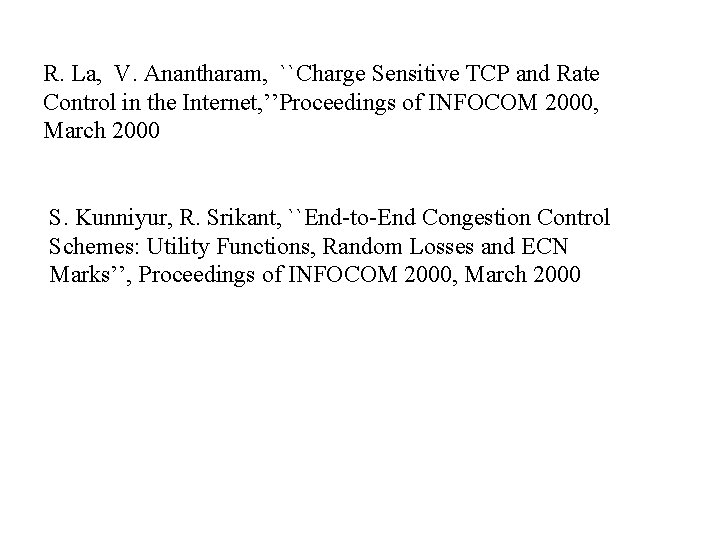R. La, V. Anantharam, ``Charge Sensitive TCP and Rate Control in the Internet, ’’Proceedings of INFOCOM 2000, March 2000 S. Kunniyur, R. Srikant, ``End-to-End Congestion Control Schemes: Utility Functions, Random Losses and ECN Marks’’, Proceedings of INFOCOM 2000, March 2000Dual based Approach Primal Maximize Ui(ri) subject to i: session i traverses link lri Cl Dual L(r, p) = i Ui(ri) - lpl( i: session i traverses link lri - Cl) = i (Ui(ri) - ri l is on session i path pl) + lpl ClD( p) = max r L (r, p) = max r i (Ui(ri) - ri l is on session i path pl) + lpl Cl = i (Ui(ri(p)) - ri (p) l is on session i path pl) + lpl Cl Ui’(ri (p)) = l is on session i path pl The optimum is attained at minp 0 D( p) Assumption: Utility functions are strictly concave. Then it turns out that the objective function Ui(ri) is strictly concave. It follows that the dual D(p) is differentiable.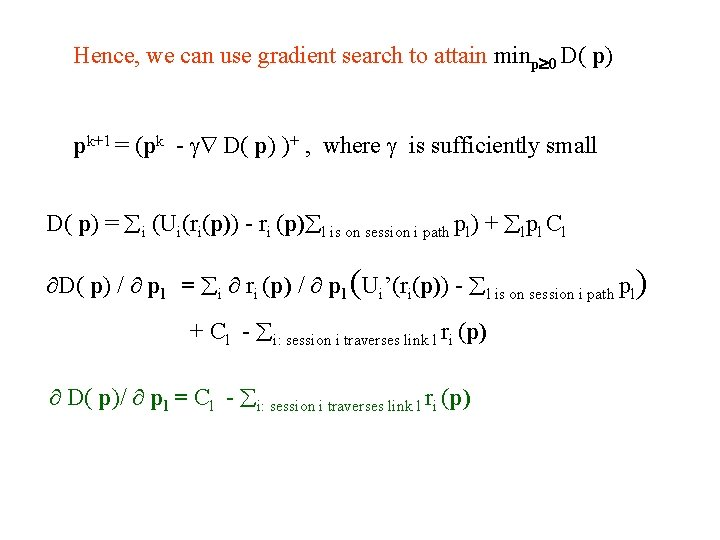Hence, we can use gradient search to attain minp 0 D( p) pk+1 = (pk - D( p) )+ , where is sufficiently small D( p) = i (Ui(ri(p)) - ri (p) l is on session i path pl) + lpl Cl D( p) / pl = i ri (p) / pl (Ui’(ri(p)) - l is on session i path pl) + Cl - i: session i traverses link l ri (p) D( p)/ pl = Cl - i: session i traverses link l ri (p)Hence, pk+1 l = (pkl - (Cl - i: session i traverses link l ri (p)))+ Also, Ui’(ri (p)) = l is on session i path pl That is, ri (p) = Ui’-1( l is on session i path pl) Initially, choose p 0 l = 0 for all links l. Update session rates as ri (0) = Ui’-1(0), for all sessions i Update link prices as p 1 l = (Cl - i: session i traverses link l ri (p))+ Update session rates again and subsequently link rates etc.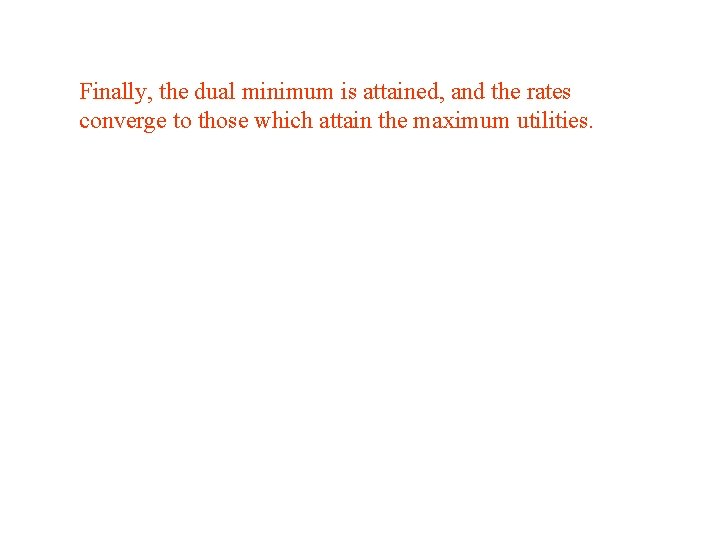Finally, the dual minimum is attained, and the rates converge to those which attain the maximum utilities.Intuitive Explanation pl is the link price. Link price update: pk+1 l = (pkl - (Cl - i: session i traverses link l ri (p)))+ New link price = Old link price - (Link Capacity – Sum of rates of sessions traversing the link) Link price increases if there is congestion, and decreases if the link bandwidth is underutilized.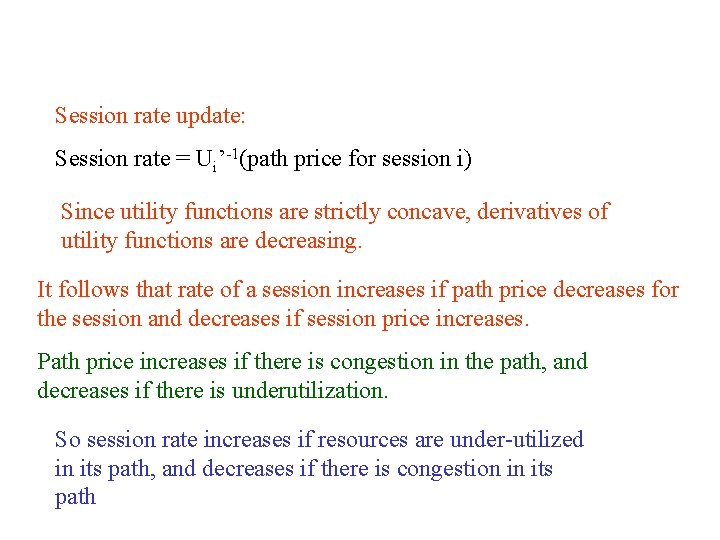Session rate update: Session rate = Ui’-1(path price for session i) Since utility functions are strictly concave, derivatives of utility functions are decreasing. It follows that rate of a session increases if path price decreases for the session and decreases if session price increases. Path price increases if there is congestion in the path, and decreases if there is underutilization. So session rate increases if resources are under-utilized in its path, and decreases if there is congestion in its path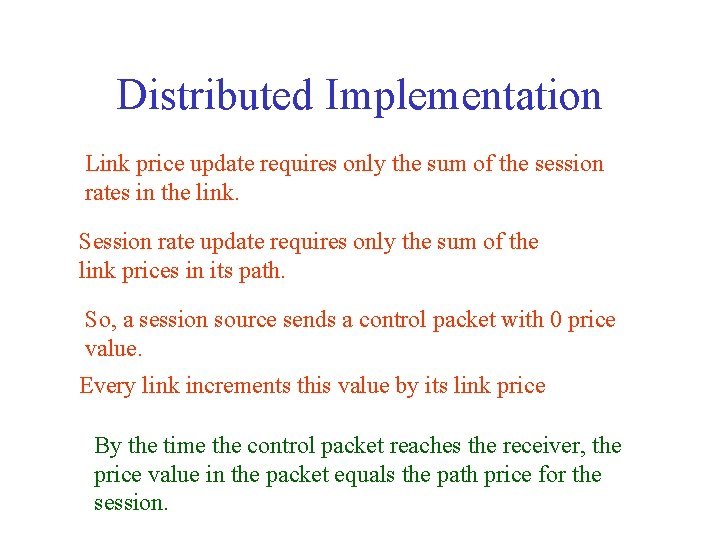Distributed Implementation Link price update requires only the sum of the session rates in the link. Session rate update requires only the sum of the link prices in its path. So, a session source sends a control packet with 0 price value. Every link increments this value by its link price By the time the control packet reaches the receiver, the price value in the packet equals the path price for the session.Session rate is updated based on the price in the control packet. Receiver communicates the rate to the session source. Links can learn the session rate from the control packet traversing towards the source from the receiver, And subsequently use these rates to update the link price. Alternatively, source sends at the rate directed by the source. Links measure the session rates from the number of incoming packets. Scheduling may play a role in the convergence for the latter!Random Early Marking A heuristic implementation of the previous optimization algorithm with one bit marking. Athuraliya, Low and Lapsley, GLOBECOM 1999 In current internet, there is a proposition to include one bit in the header of every packet for congestion notification (ECN: explicit congestion notification) If a router is congested, then it marks the bit for every packet traversing the router.When the marked packet reaches the receiver, it knows that the path is congested and asks the source to reduce the transmission rate. REM suggests that a link l mark a packet of any session with probability 1 -exp(-pl), where pl is the link price. Probability that a packet is marked is 1 - l(1 – (1 -exp(-pl))) (assuming that packet markings in different links are independent events) Probability that a packet is marked for a session i is 1 - exp (- l is on session i pathpl)This probability can be estimated from the fraction of marked packets reaching the receiver. Clearly, path price for a session can be estimated from this probability. Path price of a session = -ln(1 – fraction of marked packets) Links estimate session rates from the packet arrival rates, Links compute the link prices from these session rates Use the link prices to probabilistically mark packets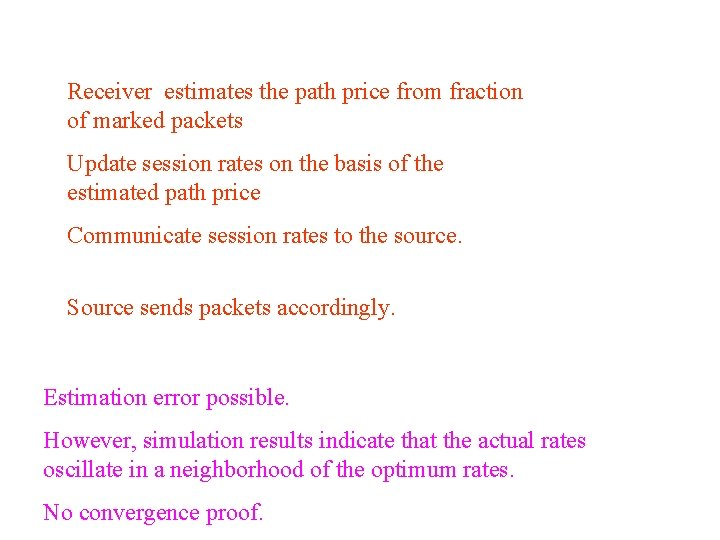Receiver estimates the path price from fraction of marked packets Update session rates on the basis of the estimated path price Communicate session rates to the source. Source sends packets accordingly. Estimation error possible. However, simulation results indicate that the actual rates oscillate in a neighborhood of the optimum rates. No convergence proof.